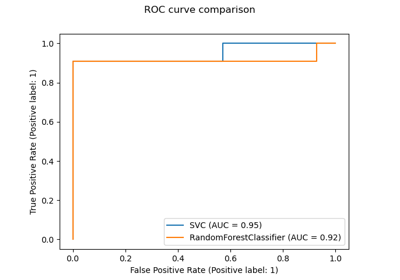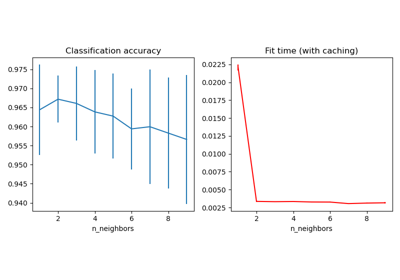# sklearn.neighbors.KNeighborsTransformer¶

class sklearn.neighbors.KNeighborsTransformer(*, mode='distance', n_neighbors=5, algorithm='auto', leaf_size=30, metric='minkowski', p=2, metric_params=None, n_jobs=1)[source]

Transform X into a (weighted) graph of k nearest neighbors.

The transformed data is a sparse graph as returned by kneighbors_graph.

Read more in the User Guide.

New in version 0.22.

Parameters:
mode{‘distance’, ‘connectivity’}, default=’distance’

Type of returned matrix: ‘connectivity’ will return the connectivity matrix with ones and zeros, and ‘distance’ will return the distances between neighbors according to the given metric.

n_neighborsint, default=5

Number of neighbors for each sample in the transformed sparse graph. For compatibility reasons, as each sample is considered as its own neighbor, one extra neighbor will be computed when mode == ‘distance’. In this case, the sparse graph contains (n_neighbors + 1) neighbors.

algorithm{‘auto’, ‘ball_tree’, ‘kd_tree’, ‘brute’}, default=’auto’

Algorithm used to compute the nearest neighbors:

Note: fitting on sparse input will override the setting of this parameter, using brute force.

leaf_sizeint, default=30

Leaf size passed to BallTree or KDTree. This can affect the speed of the construction and query, as well as the memory required to store the tree. The optimal value depends on the nature of the problem.

metricstr or callable, default=’minkowski’

Metric to use for distance computation. Default is “minkowski”, which results in the standard Euclidean distance when p = 2. See the documentation of scipy.spatial.distance and the metrics listed in distance_metrics for valid metric values.

If metric is a callable function, it takes two arrays representing 1D vectors as inputs and must return one value indicating the distance between those vectors. This works for Scipy’s metrics, but is less efficient than passing the metric name as a string.

Distance matrices are not supported.

pint, default=2

Parameter for the Minkowski metric from sklearn.metrics.pairwise.pairwise_distances. When p = 1, this is equivalent to using manhattan_distance (l1), and euclidean_distance (l2) for p = 2. For arbitrary p, minkowski_distance (l_p) is used.

metric_paramsdict, default=None

Additional keyword arguments for the metric function.

n_jobsint, default=1

The number of parallel jobs to run for neighbors search. If -1, then the number of jobs is set to the number of CPU cores.

Attributes:
effective_metric_str or callable

The distance metric used. It will be same as the metric parameter or a synonym of it, e.g. ‘euclidean’ if the metric parameter set to ‘minkowski’ and p parameter set to 2.

effective_metric_params_dict

Additional keyword arguments for the metric function. For most metrics will be same with metric_params parameter, but may also contain the p parameter value if the effective_metric_ attribute is set to ‘minkowski’.

n_features_in_int

Number of features seen during fit.

New in version 0.24.

feature_names_in_ndarray of shape (n_features_in_,)

Names of features seen during fit. Defined only when X has feature names that are all strings.

New in version 1.0.

n_samples_fit_int

Number of samples in the fitted data.

kneighbors_graph

Compute the weighted graph of k-neighbors for points in X.

RadiusNeighborsTransformer

Transform X into a weighted graph of neighbors nearer than a radius.

Examples

>>> from sklearn.datasets import load_wine
>>> from sklearn.neighbors import KNeighborsTransformer
>>> X.shape
(178, 13)
>>> transformer = KNeighborsTransformer(n_neighbors=5, mode='distance')
>>> X_dist_graph = transformer.fit_transform(X)
>>> X_dist_graph.shape
(178, 178)


Methods

 fit(X[, y]) Fit the k-nearest neighbors transformer from the training dataset. fit_transform(X[, y]) Fit to data, then transform it. get_feature_names_out([input_features]) Get output feature names for transformation. get_params([deep]) Get parameters for this estimator. kneighbors([X, n_neighbors, return_distance]) Find the K-neighbors of a point. kneighbors_graph([X, n_neighbors, mode]) Compute the (weighted) graph of k-Neighbors for points in X. set_params(**params) Set the parameters of this estimator. Compute the (weighted) graph of Neighbors for points in X.
fit(X, y=None)[source]

Fit the k-nearest neighbors transformer from the training dataset.

Parameters:
X{array-like, sparse matrix} of shape (n_samples, n_features) or (n_samples, n_samples) if metric=’precomputed’

Training data.

yIgnored

Not used, present for API consistency by convention.

Returns:
selfKNeighborsTransformer

The fitted k-nearest neighbors transformer.

fit_transform(X, y=None)[source]

Fit to data, then transform it.

Fits transformer to X and y with optional parameters fit_params and returns a transformed version of X.

Parameters:
Xarray-like of shape (n_samples, n_features)

Training set.

yIgnored

Not used, present for API consistency by convention.

Returns:
Xtsparse matrix of shape (n_samples, n_samples)

Xt[i, j] is assigned the weight of edge that connects i to j. Only the neighbors have an explicit value. The diagonal is always explicit. The matrix is of CSR format.

get_feature_names_out(input_features=None)[source]

Get output feature names for transformation.

Parameters:
input_featuresarray-like of str or None, default=None

Only used to validate feature names with the names seen in fit.

Returns:
feature_names_outndarray of str objects

Transformed feature names.

get_params(deep=True)[source]

Get parameters for this estimator.

Parameters:
deepbool, default=True

If True, will return the parameters for this estimator and contained subobjects that are estimators.

Returns:
paramsdict

Parameter names mapped to their values.

kneighbors(X=None, n_neighbors=None, return_distance=True)[source]

Find the K-neighbors of a point.

Returns indices of and distances to the neighbors of each point.

Parameters:
Xarray-like, shape (n_queries, n_features), or (n_queries, n_indexed) if metric == ‘precomputed’, default=None

The query point or points. If not provided, neighbors of each indexed point are returned. In this case, the query point is not considered its own neighbor.

n_neighborsint, default=None

Number of neighbors required for each sample. The default is the value passed to the constructor.

return_distancebool, default=True

Whether or not to return the distances.

Returns:
neigh_distndarray of shape (n_queries, n_neighbors)

Array representing the lengths to points, only present if return_distance=True.

neigh_indndarray of shape (n_queries, n_neighbors)

Indices of the nearest points in the population matrix.

Examples

In the following example, we construct a NearestNeighbors class from an array representing our data set and ask who’s the closest point to [1,1,1]

>>> samples = [[0., 0., 0.], [0., .5, 0.], [1., 1., .5]]
>>> from sklearn.neighbors import NearestNeighbors
>>> neigh = NearestNeighbors(n_neighbors=1)
>>> neigh.fit(samples)
NearestNeighbors(n_neighbors=1)
>>> print(neigh.kneighbors([[1., 1., 1.]]))
(array([[0.5]]), array([]))


As you can see, it returns [[0.5]], and [], which means that the element is at distance 0.5 and is the third element of samples (indexes start at 0). You can also query for multiple points:

>>> X = [[0., 1., 0.], [1., 0., 1.]]
>>> neigh.kneighbors(X, return_distance=False)
array([,
]...)

kneighbors_graph(X=None, n_neighbors=None, mode='connectivity')[source]

Compute the (weighted) graph of k-Neighbors for points in X.

Parameters:
Xarray-like of shape (n_queries, n_features), or (n_queries, n_indexed) if metric == ‘precomputed’, default=None

The query point or points. If not provided, neighbors of each indexed point are returned. In this case, the query point is not considered its own neighbor. For metric='precomputed' the shape should be (n_queries, n_indexed). Otherwise the shape should be (n_queries, n_features).

n_neighborsint, default=None

Number of neighbors for each sample. The default is the value passed to the constructor.

mode{‘connectivity’, ‘distance’}, default=’connectivity’

Type of returned matrix: ‘connectivity’ will return the connectivity matrix with ones and zeros, in ‘distance’ the edges are distances between points, type of distance depends on the selected metric parameter in NearestNeighbors class.

Returns:
Asparse-matrix of shape (n_queries, n_samples_fit)

n_samples_fit is the number of samples in the fitted data. A[i, j] gives the weight of the edge connecting i to j. The matrix is of CSR format.

NearestNeighbors.radius_neighbors_graph

Compute the (weighted) graph of Neighbors for points in X.

Examples

>>> X = [, , ]
>>> from sklearn.neighbors import NearestNeighbors
>>> neigh = NearestNeighbors(n_neighbors=2)
>>> neigh.fit(X)
NearestNeighbors(n_neighbors=2)
>>> A = neigh.kneighbors_graph(X)
>>> A.toarray()
array([[1., 0., 1.],
[0., 1., 1.],
[1., 0., 1.]])

set_params(**params)[source]

Set the parameters of this estimator.

The method works on simple estimators as well as on nested objects (such as Pipeline). The latter have parameters of the form <component>__<parameter> so that it’s possible to update each component of a nested object.

Parameters:
**paramsdict

Estimator parameters.

Returns:
selfestimator instance

Estimator instance.

transform(X)[source]

Compute the (weighted) graph of Neighbors for points in X.

Parameters:
Xarray-like of shape (n_samples_transform, n_features)

Sample data.

Returns:
Xtsparse matrix of shape (n_samples_transform, n_samples_fit)

Xt[i, j] is assigned the weight of edge that connects i to j. Only the neighbors have an explicit value. The diagonal is always explicit. The matrix is of CSR format.

## Examples using sklearn.neighbors.KNeighborsTransformer¶Release Highlights for scikit-learn 0.22

Release Highlights for scikit-learn 0.22Approximate nearest neighbors in TSNE

Approximate nearest neighbors in TSNECaching nearest neighbors

Caching nearest neighbors